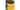### Using Python Enum and IntEnum Like An ExpertHello guys. If you use forms on your Django web applications and you want to clean model scheme, you should use enums!

## Let's start

For example, you have a product model and your visitors select something on form like status.

We consider the options as follows:

• Pending
• Published

...you have a two option. First one is complex and unmanagable. You can create tuple like below and add 'choises' attribution to CharField:

``````STATUS = (
("PENDING", "Pending"),
("PUBLISHED", "Published"),
)``````

or

``````STATUS = (
(2, "PENDING"),
(3, "PUBLISHED"),
)``````

``````class Product(models.Model):
verbose_name="Category Name", blank=True, null=True)
title = models.CharField(max_length=50, verbose_name="Product Title",
blank=True, null=True)
status = models.CharField(choices=STATUS)``````

It is correct and will work without errors. Python will save them to db as string. But it is both complex and unmanageable. Because If you want to use this field anywhere, you need to call like below because tuple is not useful for calling:

``````def example(self):
if self.status == 1:
make_something()
elif self.status == 2:
make_something()``````

I prefer using Enum or IntEnum. Firstyl we need import Enum:

``from enum import Enum, IntEnum``

Let's create our enum classes. Don't worry, we don't need create new migrations.

``````class Status(enum.Enum):
PENDING = "Pending"
PUBLISHED = "Published"``````

or (how you want to keep them)

``````class Status(enum.IntEnum):
PENDING = 2
PUBLISHED = 3``````

And your model should be below:

``````class Product(models.Model):
...
status = models.CharField(
choices=(
(x.value, x.name.title()) for x in Status),
)``````

or

``````class Product(models.Model):
...
status = models.IntegerField(
choices=(
(x.value, x.name.title()) for x in Status),
)``````

You can set a default value as I added above. How do you use Enum on functions or anywhere?

You will call enums like below:

``````def example(self):
``````def get_states(self):